# HBSE 9th Class Maths Important Questions Chapter 11 Constructions

Haryana State Board HBSE 9th Class Maths Important Questions Chapter 11 Constructions Important Questions and Answers.

## Haryana Board 9th Class Maths Important Questions Chapter 11 Constructions

Question 1.
With the help of protractor, draw an angle of 110°. Bisect it to get an angle of measure 55°. [NCERT Exemplar Problems]
Solution:
Steps of construction:
Step – I: Draw a ray BC.
Step – II: Construct ∠ABC = 110°, with the help of protractor.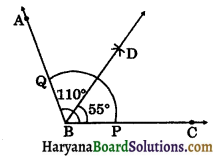Step – III: Taking B as centre and a suitable radius, draw an arc intersecting ray AB and BC at Q and P respectively.
Step – IV: Taking P and Q as the centres and radius more than $$\frac{1}{2}$$PQ, draw two arcs intersecting each other at D.
Step – V: Draw ray BD. Ray BD is required bisector of ∠ABC. On measuring with the help of the protractor, we get ∠CBD = ∠ABD = 55°.

Question 2.
Draw adjacent supplementary angles. Bisect each of the two angles. Verify that the two bisecting rays are perpendicular to each other.
Solution:
Steps of construction :
Step – I: Draw ∠AOB = 180°.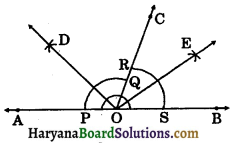Step – II: Draw ray OC such that it divides ∠AOB into two adjacent supplementary angles.
i.e., ∠AOC + ∠BOC = 180°.
Step – III: Draw the bisector OD of ∠AOC as followed by the steps in 1.
Step – IV: Draw the bisector OE of ∠BOC as followed by the steps in 1.
Verification: Since ray OD is the bisector of ∠AOC.
∴ ∠AOD = ∠COD = $$\frac{1}{2}$$∠AOC…(i)
Similarly, QE is the bisector of ∠BOC.
∴ ∠BOE = ∠COE = $$\frac{1}{2}$$∠BOC …..(ii)
Adding (i) and (ii), we get
∠COD + ∠COE = $$\frac{1}{2}$$∠AOC + $$\frac{1}{2}$$∠BOC
⇒ ∠COD + ∠COE = $$\frac{1}{2}$$(∠AOC + ∠BOC)
⇒ ∠DOE = $$\frac{1}{2}$$ × 180°
[∵ ∠AOC and ∠BOC are supplementary angles]
⇒ ∠DOE = 90°
DO ⊥ OE
Yes, bisecting rays are perpendicular to each other.Question 3.
Draw a circle whose centre is O. Draw its chord AB, draw the perpendicular bisector of line segment AB. Does it pass through the centre of the circle.
Solution:
Steps of construction:
Step – I: With the help of compass and scale draw a circle of radius 4.5 cm (say) whose centre is O.
Step – II: Draw chord AB of suitable length.
Step – III: Taking A as the centre and radius more than $$\frac{1}{2}$$AB draw two arcs one on each side of AB.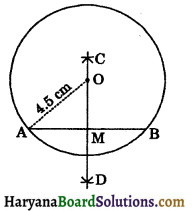Step – IV: Taking B as the centre and same radius as before, draw two arcs intersecting previous arc at C and D respectively.
Step – V: Join CD, intersecting AB at M. So, CD is the perpendicular bisector of AB. Yes, perpendicular bisector CD passes through the centre of the circle.

Question 4.
Draw a circle whose centre is O. Draw its two chords PQ and QR such that PQ ≠ RS. Do they intersect at point O?
Solution:
Steps of construction:
Step – I: Draw a circle of suitable radius whose centre is O.
Step – II: Draw two chords PQ and QR of suitable lengths.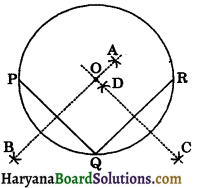Step – III: Draw the perpendicular bisector AB of chord PQ as followed by the steps in 3.
Step – IV: Similarly draw the perpendicular bisector CD of chord QR.
Step – V: AB and CD intersect each other at point O.
Yes, perpendicular bisectors of chord PQ and chord QR intersect each other at point O.

Question 5.
Draw a line segment of length 10 cm with the help of ruler and compass to obtain a length of 7.5 cm.
Solution:
Steps of construction :
Step – I: Draw a line segment of length 10 cm.
Step – II: Draw the perpendicular bisector PQ of line AB as followed by the steps in 3 intersecting AB at M.
∴ AM = MB = $$\frac{10}{2}$$ = 5 cm.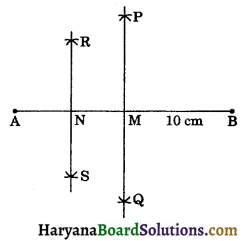Step – III: Similarly, draw the perpendicular bisector of AM intersecting AM at N.
∴ AN = NM = $$\frac{5}{2}$$ = 2.5 cm
Now, BN = BM + NM
⇒ BN = 5 cm + 2.5 cm
⇒ BN = 7.5 cm
Hence, we obtain BN of length 7.5 cm.Question 6.
Using ruler and compass only, construct the following angles :
(a) 120° (b) 150° (c) 37$$\frac{1^{\circ}}{2}$$
Solution:
(a) Steps of construction:
Step – I: Draw a ray AB with initial point A.
Step – II: Taking A as centre and a suitable radius, draw an arc intersecting AB at P.
Step – III: Taking P as centre and same radius as before, draw an arc intersecting the previous arc at Q.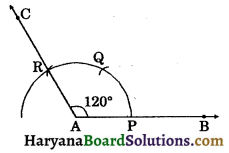Step – IV: Taking Q as centre and same radius as before, draw an arc intersecting the previous arc at R.
Step – V: Join AR and produced it to C. Then ∠CAB = 120° is required angle.

(b) Steps of construction:
Step – I: Draw a ray AB with initial point A.
Step – II: Taking A as centre and a suitable radius, draw an arc intersecting AB at P.
Step – III: Taking P as centre and same radius as before, draw an arc intersecting previous arc at Q.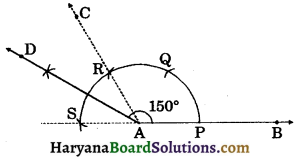Step – IV: Taking Q as centre and same radius as before, draw an arc intersecting previous arc at R. Join AR and produced it to C. Then ZCAB = 120°.
Step – V: Taking R as centre and same radius as before, draw an arc intersecting previous arc at S. Join AS. Then ∠CAS = 180° – 120° = 60°.
Step – VI: Draw bisector AD of ∠CAS.
∴ ∠CAD = $$\frac{1}{2}$$ × ∠CAS
⇒ ∠CAD = $$\frac{1}{2}$$ × 60° = 30°
∠DAB = 120° + 30° = 150°.
Hence, ∠DAB = 150° is the required angle.

(c) Steps of construction:
Step – I: Draw a ray AB with initial point A.
Step – II: Draw ∠CAB = 60° as followed by the steps in Construction 11.3.
Step – III: Draw ∠DAB = 90° as followed by the steps in 1. of Ex. 11:1. Then
∠DAC = 90° – 60° = 30°.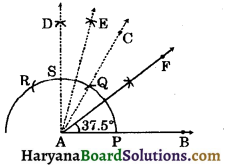Step – IV: Draw bisector AE of ∠DAC.
∴ ∠DAE = ∠CAE = $$\frac{30^{\circ}}{2}$$ = 15°
∠EAB = 90° – ∠DAE
⇒ ∠EAB = 90° – 15° = 75°.
Step – V: Draw the bisector AF of ∠EAB.
∴ ∠FAB = $$\frac{1}{2}$$∠EAB
⇒ ∠FAB = $$\frac{75^{\circ}}{2}$$ = 37$$\frac{1^{\circ}}{2}$$
Hence, ∠FAB = 37$$\frac{1^{\circ}}{2}$$ is the required angle.

Question 7.
Construct an equilateral triangle, if one of its altitude is 3.2 cm. [NCERT Exemplar Problems]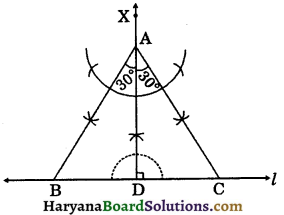Solution:
Steps of construction:
Step – I: Draw a line l.
Step – II: Mark any point D on the line l.
Step – III: At point D, draw DX ⊥ l and cut off AD = 3.2 cm.
Step – IV: Construct ∠DAB = 30° and ∠DAC = 30° intersecting l at B and C respectively.
Then ABC is the required triangle.Question 8.
Construct a triangle ABC such that AB = 6 cm, BC = 6 cm and median CM = 4.3 cm.
Solution:
Steps of construction:
Step – I: Draw a line segment AB = 6 cm.
Step – II: Draw the bisector of AB intersecting AB at M.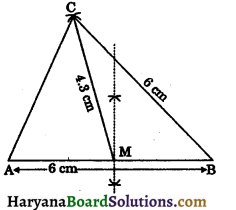Step – III: Taking M as the centre and radius 4.3 cm draw an arc.
Step – IV: Taking B as the centre and radius 6 cm, draw another arc which intersects the previous arc at point C.
Step – V: Join AC and BC, then ABC is the required triangle.

Question 9.
Construct an isosceles triangle whose base 6 cm and vertical angle is 60°.
Solution:
Steps of construction:
Step – I: Draw a line segment BC = 6 cm.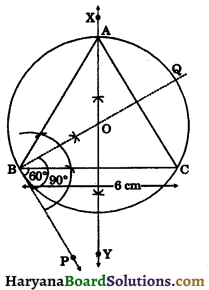Step – II: Construct ∠CBP = 60°, below the line segment BC.
Step – III: Construct ∠PBQ = 90°.
Step – IV: Draw the perpendicular bisector XY of BC, intersecting BQ at O.
Step – V: Taking O as the centre and radius OB, draw a circle intersecting XY at A.
Step – VI: Join AB and AC, then ABC is the required triangle.

Question 10.
Construct right angled triangle whose hypotenuse measures 7 cm and the length of one of whose sides containing the right angle is 5 m.
Solution:
Steps of construction:
Step – I: Draw a line segment BC = 7 cm.
Step – II: Draw the perpendicular bisector of BC intersecting BC at O.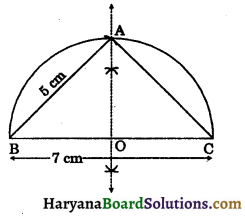Step – III: Taking O as centre and radius OB, draw a semi-circle on BC.
Step – IV: Taking B as the centre and radius equal to 5 cm, draw an arc, interscting the semicircle at A.
Step – V: Join AB and AC. Then ABC is the required triangle.Question 11.
Construct a right angled triangle in which ∠B = 90°, hypotenuse AC = 13 cm and the sum of the remaining sides AB and BC is 17 cm.
Solution:
Sum of the remaining two sides = 17 cm (given)
Let one of its side be x, then other its side is (17 – x) cm
hypotenuse = 13 cm
In right triangle, we have
(hypotenuse)2 = (one side)2 + (other side)2
⇒ 132 = x2 + (17 – x)2
⇒ 169 = x2 + 289 + 32 – 34x
⇒ 0 = 2x2 – 34x + 289 – 169
⇒ 2x2 – 34x + 120 = 0
⇒ x2 – 17x + 60 = 0
⇒ x2 – 12x – 5x + 60 = 0
⇒ x(x – 12) -5 (x – 12) = 0
⇒ (x – 12) (x – 5) = 0
⇒ x – 12 = 0 or x – 5 = 0
x = 12 or x = 5
So, sides are 12 cm and 17 – 12 = 5 cm.
So, we construct a right triangle in which ∠B = 90°, hypotenuse (AC) = 13 cm and other sides AB = 12 cm and BC = 5 cm.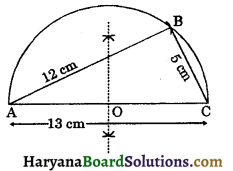Steps of construction:
Step – I: Draw a line segment AC = 13 cm.
Step – II: Draw the perpendicular bisector of AC intersecting AC at O.
Step – III: Taking O as the centre and radius AO, draw a semicircle on AC.
Step – IV: Taking A as the centre and radius equal to 12 cm, draw an arc interscting the semicircle at B.
Step – V: Join AB and BC. The ABC is the required triangle.

Question 12.
Construct a triangle ABC whose perimeter is 12 cm and sides are in the ratio 3 : 4 : 5.
Solution:
Steps of construction:
Step – I: Draw a line segment PQ = 12 cm (= perimeter).
Step – II : At the point Q, draw an acute ∠PQX with PQ.
Step – III: Divide QX into 3 + 4 + 5 = 12 equal parts.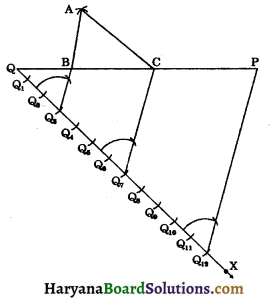Step – IV: Join Q12 to P.
Step – V: From Q3, draw BQ3 || PQ12 intersecting PQ at B.
Step – VI: From Q7, draw CQ7 || PQ12 intersecting PQ at C.
Step – VII: Taking B as centre and radius as QB, draw an arc.
Step – VIII: Taking C as the centre and radius as CP, draw another arc, which intersects the previous arc at A.
Step – IX: Join AB and AC, then ABC is the required triangle.

Question 13.
Construct a triangle having its perimeter 11.5 cm and ratio of the angles as 3 : 4 : 5.
Solution:
Ratio of angles = 3 : 4 : 5
Sum of ratios = 3 + 4 + 5 = 12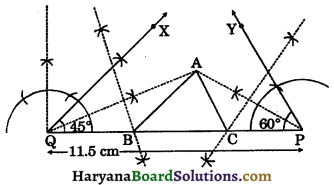Ist angle = $$\frac{13}{2}$$ × 180°
[∵ Sum of angles of a triangle is 180°]
⇒ Ist angle = 45°
⇒ IInd angle = $$\frac{4}{12}$$ × 180°
⇒ IInd angle = 60°
IIIrd angle = $$\frac{5}{12}$$ × 180°
⇒ IIIrd angle = 75°.
Let base angles be 45° and 60° and perimeter of triangle is 11.5 cm (given).
Now we construct a ΔABC as follows:
Steps of construction:
Step – I: Draw a line segment PQ = 11.5 cm.
Step – II: At the point Q construct ∠PQX = 45° and at the point P construct ∠QPY = 60°.
Step – III: Draw the bisectors of ∠PQX and ∠QPY intersecting at A.
Stop – IV: Draw the perpendicular bisectors of AQ and AP intersecting PQ at B and C respectively.
Step – V: Join AB and AC, then ABC is the required triangle.Question 14.
Construct a triangle ABC, the lengths of whose medians are 5 cm, 5 cm and 6 cm. Measure the length of sides of ΔABC.
Solution:
In a ΔABC, let medians AD = 5 cm, BE = 6 cm and CF = 5 cm. We need to construct a ΔABC.
Steps of construction:
Step – I: Construct a triangle AXD with sides AX = 5 cm, AD = 5 cm and XD = 6 cm.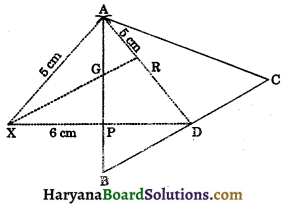Step – II: Draw the medians AP and XR intersecting each other at point G.
Step – III: Extend GP to B such that GP = PB.
Step – IV: Join BD and produced to it C such that BD = CD.
Step – V: Join A to C, then ABC is the required triangle and AD its median.
On measuring the sides of ΔABC, we get AB = 5.95 cm, AC = 6.5 cm, BC = 6.5 cm.

Question 15.
Construct a rhombus whose diagonals are 4 cm and 6 cm in lengths. [NCERT Exemplar Problems]
Solution:
We know that, diagonals of a rhombusbisect each other at right angles.
Let AC and BD are diagonals of a rhombus and bisect each other at O.
Steps of construction:
Step – I: Draw diagonal AC of length 6 cm.
Step – II: Draw perpendicular bisector XY of AC intersecting AC at O.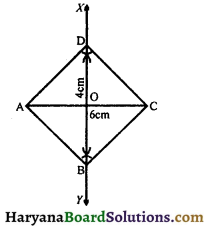Step – III: From O, cuts OB = OD = 2 cm.
Step – IV: Join AB, BC, CD and DA. Then ABCD is the required rhombus.

Multiple Choice Questions

Choose the correct option in each of the following:

Question 1.
Which of the following angle cannot be constructed using ruler and compass only: [NCERT Exemplar Problems]
(a) 22°$$\frac{1^{\circ}}{2}$$
(b) 67$$\frac{1^{\circ}}{2}$$
(c) 40°
(d) 37$$\frac{1^{\circ}}{2}$$
(c) 40°

Question 2.
Which of the following angle cannot be constructed using ruler and compass only:
(a) 60°
(b) 150°
(c) 65°
(d) 22$$\frac{1^{\circ}}{2}$$
(c) 65°Question 3.
Which of the following angle can be constructed using ruler and compass only:
(a) 85°
(b) 115°
(c) 47$$\frac{1^{\circ}}{2}$$
(d) 75°
(d) 75°

Question 4.
Which of the following angle can be constructed using ruler and compass only:
(a) 82$$\frac{1^{\circ}}{2}$$
(b) 92°
(c) 100°
(d) 122$$\frac{1^{\circ}}{2}$$
(a) 82$$\frac{1^{\circ}}{2}$$

Question 5.
It is not possible to construct a triangle when its sides are :
(a) 4 cm, 2 cm and 6 cm
(b) 7 cm, 4 cm and 5 cm
(c) 4 cm, 5 cm and 8 cm
(d) 5 cm, 12 cm and 13 cm
(a) 4 cm, 2 cm and 6 cm

Question 6.
It is possible to construct a triangle when its sides are :
(a) 5 cm, 6 cm and 13 cm
(b) 5 cm, 7 cm and 12 cm
(c) 6 cm, 4 cm and 9 cm
(d) 3 cm, 5 cm and 10 cm
(c) 6 cm, 4 cm and 9 cmQuestion 7.
It is possible to construct a triangle when its angles are :
(a) 40°, 55°, 60°
(b) 55°, 60°, 65°
(c) 50°, 70°, 80°
(d) 67°, 63°, 51°
(b) 55°, 60°, 65°

Question 8.
The construction of ΔABC in which BC = 8 cm, ∠C = 50° is possible when (AC + BC) is :
(a) 10 cm
(b) 8 cm
(c) 7 cm
(d) 6 cm
(a) 10 cm

Question 9.
The construction of ΔABC in which AB = 5 cm, ∠B = 70° is not possible when (BC + AC) is :
(a) 7 cm
(b) 8.5 cm
(c) 4.5 cm
(d) 9 cm
(c) 4.5 cm

Question 10.
The construction of a triangle ABC in which AB = 4 cm, ∠A = 60° is not possible when difference of BC and AC is equal to: [NCERT Exemplar Problems]
(a) 3.5 cm
(b) 4.5 cm
(c) 3 cm
(d) 2.5 cm
(b) 4.5 cmQuestion 11.
The construction of a triangle ABC, given that BC = 6 cm, ∠B = 45° is not possible when difference of AB and AC is equal to : [NCERT Exemplar Problems]
(a) 6.9 cm
(b) 5.2 cm
(c) 5.0 cm
(d) 4.0 cm